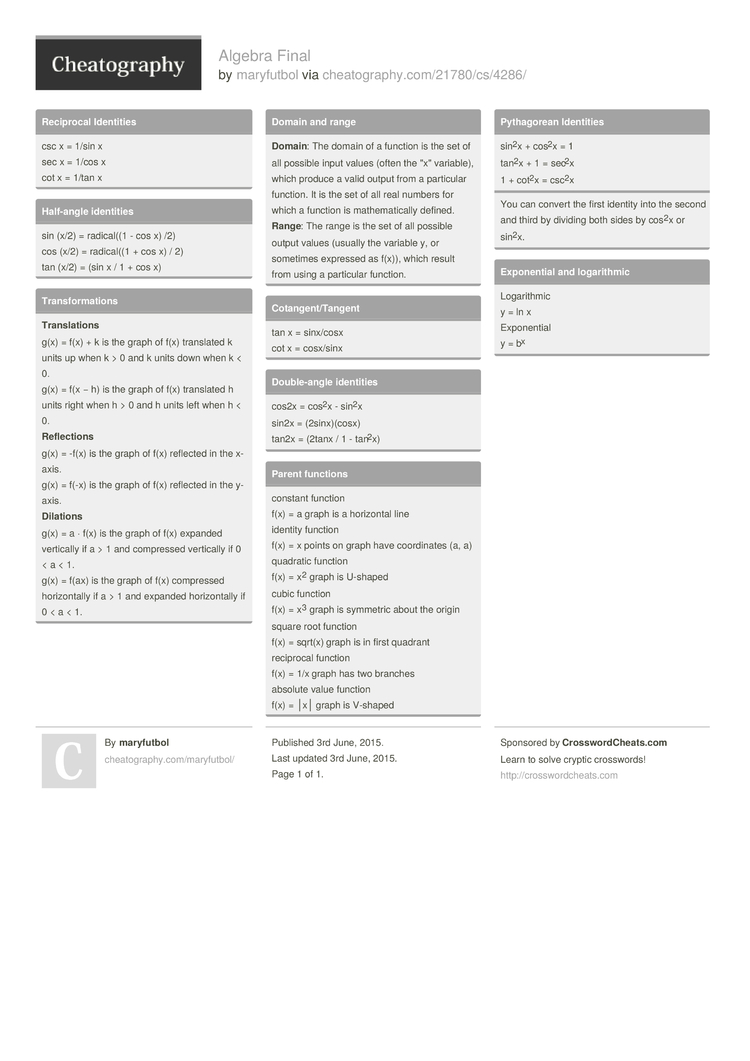Show Menu

# Algebra Final by maryfutbol

### Reciprocal Identities

 csc x = 1/sin x sec x = 1/cos x cot x = 1/tan x

### Half-angle identities

 sin (x/2) = radical((1 - cos x) /2) cos (x/2) = radical((1 + cos x) / 2) tan (x/2) = (sin x / 1 + cos x)

### Transf­orm­ations

 Tran­sla­tions g(x) = f(x) + k is the graph of f(x) translated k units up when k > 0 and k units down when k < 0. g(x) = f(x − h) is the graph of f(x) translated h units right when h > 0 and h units left when h < 0. Refl­ect­ions g(x) = -f(x) is the graph of f(x) reflected in the x-axis. g(x) = f(-x) is the graph of f(x) reflected in the y-axis. Dila­tions g(x) = a · f(x) is the graph of f(x) expanded vertically if a > 1 and compressed vertically if 0 < a < 1. g(x) = f(ax) is the graph of f(x) compressed horizo­ntally if a > 1 and expanded horizo­ntally if 0 < a < 1.

### Domain and range

 Doma­in: The domain of a function is the set of all possible input values (often the "­x" variable), which produce a valid output from a particular function. It is the set of all real numbers for which a function is mathem­ati­cally defined. Range: The range is the set of all possible output values (usually the variable y, or sometimes expressed as f(x)), which result from using a particular function.

### Cotang­ent­/Ta­ngent

 tan x = sinx/cosx cot x = cosx/sinx

### Double­-angle identities

 cos2x = cos2x - sin2x sin2x = (2sinx­)(cosx) tan2x = (2tanx / 1 - tan2x)

### Parent functions

 constant function f(x) = a graph is a horizontal line identity function f(x) = x points on graph have coordi­nates (a, a) quadratic function f(x) = x2 graph is U-shaped cubic function f(x) = x3 graph is symmetric about the origin square root function f(x) = sqrt(x) graph is in first quadrant reciprocal function f(x) = 1/x graph has two branches absolute value function f(x) = │x│ graph is V-shaped

### Pythag­orean Identities

 sin2x + cos2x = 1 tan2x + 1 = sec2x 1 + cot2x = csc2x
You can convert the first identity into the second and third by dividing both sides by cos2x or sin2x.

### Expone­ntial and logari­thmic

 Logari­thmic y = ln x Expone­ntial y = bx

## Download the Algebra Final1 Page
//media.cheatography.com/storage/thumb/maryfutbol_algebra-final.750.jpg

PDF (recommended)

Alternative Downloads

## Comments

No comments yet. Add yours below!

## Add a Comment

Your Comment

Please enter your name.

Please enter your email address

Please enter your Comment.